Exponential FunctionsFigure 1. Electron micrograph of E.Coli bacteria (credit: “Mattosaurus,” Wikimedia Commons)

Focus in on a square centimeter of your skin. Look closer. Closer still. If you could look closely enough, you would see hundreds of thousands of microscopic organisms. They are bacteria, and they are not only on your skin, but in your mouth, nose, and even your intestines. In fact, the bacterial cells in your body at any given moment outnumber your own cells. But that is no reason to feel bad about yourself. While some bacteria can cause illness, many are healthy and even essential to the body.

Bacteria commonly reproduce through a process called binary fission, during which one bacterial cell splits into two. When conditions are right, bacteria can reproduce very quickly. Unlike humans and other complex organisms, the time required to form a new generation of bacteria is often a matter of minutes or hours, as opposed to days or years.

For simplicity’s sake, suppose we begin with a culture of one bacterial cell that can divide every hour. The table below shows the number of bacterial cells at the end of each subsequent hour. We see that the single bacterial cell leads to over one thousand bacterial cells in just ten hours! And if we were to extrapolate the table to twenty-four hours, we would have over 16 million!

 Hour 0 1 2 3 4 5 6 7 8 9 10 Bacteria 1 2 4 8 16 32 64 128 256 512 1024

Exponential Functions

In this chapter, we will explore exponential functions, which can be used for, among other things, modeling growth patterns such as those found in bacteria. We will also investigate logarithmic functions, which are closely related to exponential functions. Both types of functions have numerous real-world applications when it comes to modeling and interpreting data.

LEARNING OBJECTIVES

By the end of this lesson, you will be able to:

• Evaluate exponential functions.
• Find the equation of an exponential function.
• Use compound interest formulas.
• Evaluate exponential functions with base e.

India is the second most populous country in the world with a population of about 1.25 billion people in 2013. The population is growing at a rate of about 1.2% each year. If this rate continues, the population of India will exceed China’s population by the year 2031. When populations grow rapidly, we often say that the growth is “exponential,” meaning that something is growing very rapidly. To a mathematician, however, the term exponential growth has a very specific meaning. In this section, we will take a look at exponential functions, which model this kind of rapid growth.

Evaluate Exponential Functions

Recall that the base of an exponential function must be a positive real number other than 1. Why do we limit the base b to positive values? To ensure that the outputs will be real numbers. Observe what happens if the base is not positive:

• Let = –9 and $x=\frac{1}{2}$. Then $f\left(x\right)=f\left(\frac{1}{2}\right)={\left(-9\right)}^{\frac{1}{2}}=\sqrt{-9}$, which is not a real number.

Why do we limit the base to positive values other than 1? Because base 1 results in the constant function. Observe what happens if the base is 1:

• Let = 1. Then $f\left(x\right)={1}^{x}=1$ for any value of x.

To evaluate an exponential function with the form $f\left(x\right)={b}^{x}$, we simply substitute x with the given value, and calculate the resulting power. For example:

Let $f\left(x\right)={2}^{x}$. What is $f\left(3\right)$?

$\begin{cases}f\left(x\right)\hfill & ={2}^{x}\hfill & \hfill \\ f\left(3\right)\hfill & ={2}^{3}\text{ }\hfill & \text{Substitute }x=3.\hfill \\ \hfill & =8\text{ }\hfill & \text{Evaluate the power}\text{.}\hfill \end{cases}$

To evaluate an exponential function with a form other than the basic form, it is important to follow the order of operations. For example:

Let $f\left(x\right)=30{\left(2\right)}^{x}$. What is $f\left(3\right)$?

$\begin{cases}f\left(x\right)\hfill & =30{\left(2\right)}^{x}\hfill & \hfill \\ f\left(3\right)\hfill & =30{\left(2\right)}^{3}\hfill & \text{Substitute }x=3.\hfill \\ \hfill & =30\left(8\right)\text{ }\hfill & \text{Simplify the power first}\text{.}\hfill \\ \hfill & =240\hfill & \text{Multiply}\text{.}\hfill \end{cases}$

Note that if the order of operations were not followed, the result would be incorrect:

$f\left(3\right)=30{\left(2\right)}^{3}\ne {60}^{3}=216,000$

Example 1: Evaluating Exponential Functions

Let $f\left(x\right)=5{\left(3\right)}^{x+1}$. Evaluate $f\left(2\right)$ without using a calculator.

Solution

Follow the order of operations. Be sure to pay attention to the parentheses.

$\begin{cases}f\left(x\right)\hfill & =5{\left(3\right)}^{x+1}\hfill & \hfill \\ f\left(2\right)\hfill & =5{\left(3\right)}^{2+1}\hfill & \text{Substitute }x=2.\hfill \\ \hfill & =5{\left(3\right)}^{3}\hfill & \text{Add the exponents}.\hfill \\ \hfill & =5\left(27\right)\hfill & \text{Simplify the power}\text{.}\hfill \\ \hfill & =135\hfill & \text{Multiply}\text{.}\hfill \end{cases}$

Try It 1

Let $f\left(x\right)=8{\left(1.2\right)}^{x - 5}$. Evaluate $f\left(3\right)$ using a calculator. Round to four decimal places.

Solution

Because the output of exponential functions increases very rapidly, the term “exponential growth” is often used in everyday language to describe anything that grows or increases rapidly. However, exponential growth can be defined more precisely in a mathematical sense. If the growth rate is proportional to the amount present, the function models exponential growth.

A General Note: Exponential Growth

A function that models exponential growth grows by a rate proportional to the amount present. For any real number x and any positive real numbers and b such that $b\ne 1$, an exponential growth function has the form

$\text{ }f\left(x\right)=a{b}^{x}$

where

• a is the initial or starting value of the function.
• b is the growth factor or growth multiplier per unit x.

In more general terms, we have an exponential function, in which a constant base is raised to a variable exponent. To differentiate between linear and exponential functions, let’s consider two companies, A and B. Company A has 100 stores and expands by opening 50 new stores a year, so its growth can be represented by the function $A\left(x\right)=100+50x$. Company B has 100 stores and expands by increasing the number of stores by 50% each year, so its growth can be represented by the function $B\left(x\right)=100{\left(1+0.5\right)}^{x}$.

A few years of growth for these companies are illustrated below.

Year, x Stores, Company A Stores, Company B
0 100 + 50(0) = 100 100(1 + 0.5)0 = 100
1 100 + 50(1) = 150 100(1 + 0.5)1 = 150
2 100 + 50(2) = 200 100(1 + 0.5)2 = 225
3 100 + 50(3) = 250 100(1 + 0.5)3 = 337.5
x A(x) = 100 + 50x B(x) = 100(1 + 0.5)x

The graphs comparing the number of stores for each company over a five-year period are shown in below. We can see that, with exponential growth, the number of stores increases much more rapidly than with linear growth.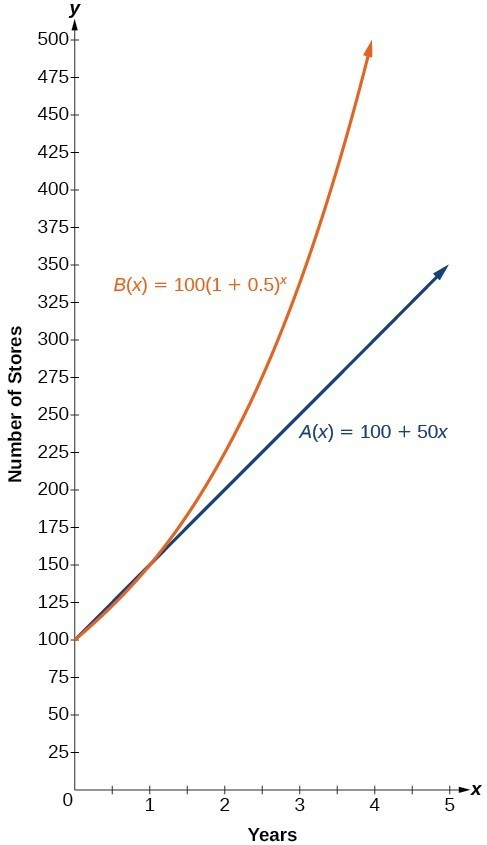Figure 2. The graph shows the numbers of stores Companies A and B opened over a five-year period.

Notice that the domain for both functions is $\left[0,\infty \right)$, and the range for both functions is $\left[100,\infty \right)$. After year 1, Company B always has more stores than Company A.

Now we will turn our attention to the function representing the number of stores for Company B, $B\left(x\right)=100{\left(1+0.5\right)}^{x}$. In this exponential function, 100 represents the initial number of stores, 0.50 represents the growth rate, and $1+0.5=1.5$ represents the growth factor. Generalizing further, we can write this function as $B\left(x\right)=100{\left(1.5\right)}^{x}$, where 100 is the initial value, 1.5 is called the base, and x is called the exponent.

Example 2: Evaluating a Real-World Exponential Model

At the beginning of this section, we learned that the population of India was about 1.25 billion in the year 2013, with an annual growth rate of about 1.2%. This situation is represented by the growth function $P\left(t\right)=1.25{\left(1.012\right)}^{t}$, where t is the number of years since 2013. To the nearest thousandth, what will the population of India be in 2031?

Solution

To estimate the population in 2031, we evaluate the models for = 18, because 2031 is 18 years after 2013. Rounding to the nearest thousandth,

$P\left(18\right)=1.25{\left(1.012\right)}^{18}\approx 1.549$

There will be about 1.549 billion people in India in the year 2031.

Try It 2

The population of China was about 1.39 billion in the year 2013, with an annual growth rate of about 0.6%. This situation is represented by the growth function $P\left(t\right)=1.39{\left(1.006\right)}^{t}$, where t is the number of years since 2013. To the nearest thousandth, what will the population of China be for the year 2031? How does this compare to the population prediction we made for India in Example 2?

Solution

Find the Equation of an Exponential Function

In the previous examples, we were given an exponential function, which we then evaluated for a given input. Sometimes we are given information about an exponential function without knowing the function explicitly. We must use the information to first write the form of the function, then determine the constants a and b, and evaluate the function.

How To: Given two data points, write an exponential model.

1. If one of the data points has the form $\left(0,a\right)$, then a is the initial value. Using a, substitute the second point into the equation $f\left(x\right)=a{\left(b\right)}^{x}$, and solve for b.
2. If neither of the data points have the form $\left(0,a\right)$, substitute both points into two equations with the form $f\left(x\right)=a{\left(b\right)}^{x}$. Solve the resulting system of two equations in two unknowns to find a and b.
3. Using the a and b found in the steps above, write the exponential function in the form $f\left(x\right)=a{\left(b\right)}^{x}$.

Example 3: Writing an Exponential Model When the Initial Value Is Known

In 2006, 80 deer were introduced into a wildlife refuge. By 2012, the population had grown to 180 deer. The population was growing exponentially. Write an algebraic function N(t) representing the population N of deer over time t.

Solution

We let our independent variable t be the number of years after 2006. Thus, the information given in the problem can be written as input-output pairs: (0, 80) and (6, 180). Notice that by choosing our input variable to be measured as years after 2006, we have given ourselves the initial value for the function, = 80. We can now substitute the second point into the equation $N\left(t\right)=80{b}^{t}$ to find b:

$\begin{cases}N\left(t\right)\hfill & =80{b}^{t}\hfill & \hfill \\ 180\hfill & =80{b}^{6}\hfill & \text{Substitute using point }\left(6, 180\right).\hfill \\ \frac{9}{4}\hfill & ={b}^{6}\hfill & \text{Divide and write in lowest terms}.\hfill \\ b\hfill & ={\left(\frac{9}{4}\right)}^{\frac{1}{6}}\hfill & \text{Isolate }b\text{ using properties of exponents}.\hfill \\ b\hfill & \approx 1.1447 & \text{Round to 4 decimal places}.\hfill \end{cases}$

NOTE: Unless otherwise stated, do not round any intermediate calculations. Then round the final answer to four places for the remainder of this section.

The exponential model for the population of deer is $N\left(t\right)=80{\left(1.1447\right)}^{t}$. (Note that this exponential function models short-term growth. As the inputs gets large, the output will get increasingly larger, so much so that the model may not be useful in the long term.)

We can graph our model to observe the population growth of deer in the refuge over time. Notice that the graph below passes through the initial points given in the problem, $\left(0,\text{ 8}0\right)$ and $\left(\text{6},\text{ 18}0\right)$. We can also see that the domain for the function is $\left[0,\infty \right)$, and the range for the function is $\left[80,\infty \right)$.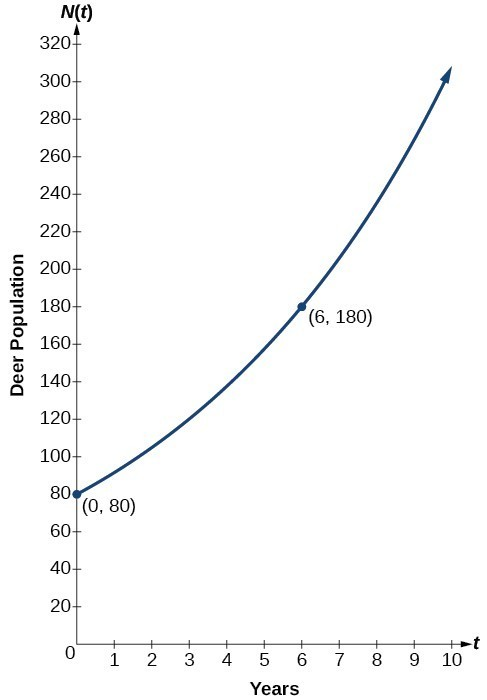Figure 3. Graph showing the population of deer over time, $N\left(t\right)=80{\left(1.1447\right)}^{t}$, t years after 2006

Try It 3

A wolf population is growing exponentially. In 2011, 129 wolves were counted. By 2013 the population had reached 236 wolves. What two points can be used to derive an exponential equation modeling this situation? Write the equation representing the population N of wolves over time t.

Solution

Example 4: Writing an Exponential Model When the Initial Value is Not Known

Find an exponential function that passes through the points $\left(-2,6\right)$ and $\left(2,1\right)$.

Solution

Because we don’t have the initial value, we substitute both points into an equation of the form $f\left(x\right)=a{b}^{x}$, and then solve the system for a and b.

• Substituting $\left(-2,6\right)$ gives $6=a{b}^{-2}$
• Substituting $\left(2,1\right)$ gives $1=a{b}^{2}$

Use the first equation to solve for a in terms of b: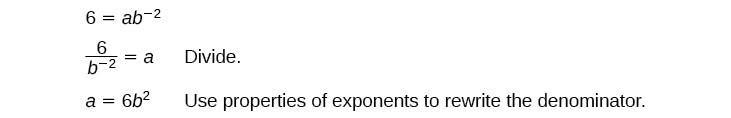Substitute a in the second equation, and solve for b: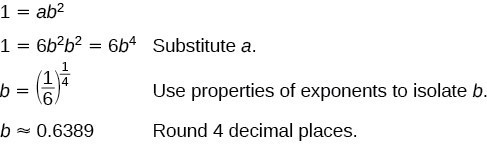Use the value of b in the first equation to solve for the value of a: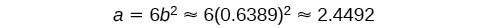Thus, the equation is $f\left(x\right)=2.4492{\left(0.6389\right)}^{x}$.

We can graph our model to check our work. Notice that the graph below passes through the initial points given in the problem, $\left(-2,\text{ 6}\right)$ and $\left(2,\text{ 1}\right)$. The graph is an example of an exponential decay function.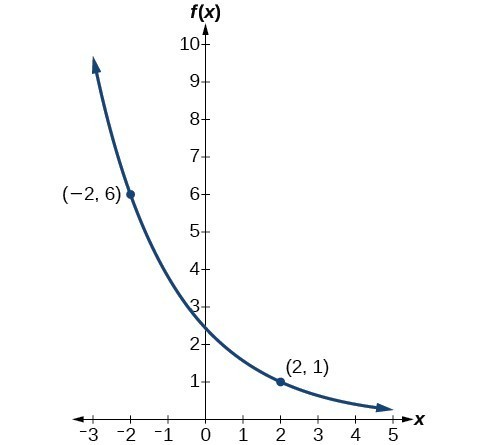Figure 4. The graph of $f\left(x\right)=2.4492{\left(0.6389\right)}^{x}$ models exponential decay.

Try It 4

Given the two points $\left(1,3\right)$ and $\left(2,4.5\right)$, find the equation of the exponential function that passes through these two points.

Solution

Q & A

Do two points always determine a unique exponential function?

Yes, provided the two points are either both above the x-axis or both below the x-axis and have different x-coordinates. But keep in mind that we also need to know that the graph is, in fact, an exponential function. Not every graph that looks exponential really is exponential. We need to know the graph is based on a model that shows the same percent growth with each unit increase in x, which in many real world cases involves time.

How To: Given the graph of an exponential function, write its equation.

1. First, identify two points on the graph. Choose the y-intercept as one of the two points whenever possible. Try to choose points that are as far apart as possible to reduce round-off error.
2. If one of the data points is the y-intercept $\left(0,a\right)$ , then a is the initial value. Using a, substitute the second point into the equation $f\left(x\right)=a{\left(b\right)}^{x}$, and solve for b.
3. If neither of the data points have the form $\left(0,a\right)$, substitute both points into two equations with the form $f\left(x\right)=a{\left(b\right)}^{x}$. Solve the resulting system of two equations in two unknowns to find a and b.
4. Write the exponential function, $f\left(x\right)=a{\left(b\right)}^{x}$.

Example 5: Writing an Exponential Function Given Its Graph

Find an equation for the exponential function graphed in Figure 5.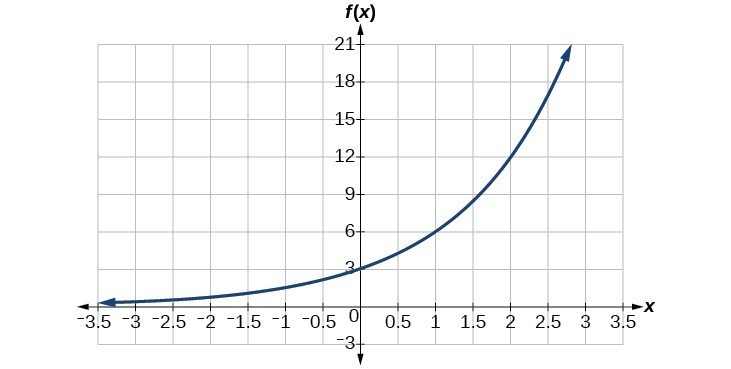Figure 5

Solution

We can choose the y-intercept of the graph, $\left(0,3\right)$, as our first point. This gives us the initial value, $a=3$. Next, choose a point on the curve some distance away from $\left(0,3\right)$ that has integer coordinates. One such point is $\left(2,12\right)$.

$\begin{cases}y=a{b}^{x}& \text{Write the general form of an exponential equation}. \\ y=3{b}^{x} & \text{Substitute the initial value 3 for }a. \\ 12=3{b}^{2} & \text{Substitute in 12 for }y\text{ and 2 for }x. \\ 4={b}^{2} & \text{Divide by 3}. \\ b=\pm 2 & \text{Take the square root}.\end{cases}$

Because we restrict ourselves to positive values of b, we will use = 2. Substitute a and b into the standard form to yield the equation $f\left(x\right)=3{\left(2\right)}^{x}$.

Try It 5

Find an equation for the exponential function graphed in Figure 6.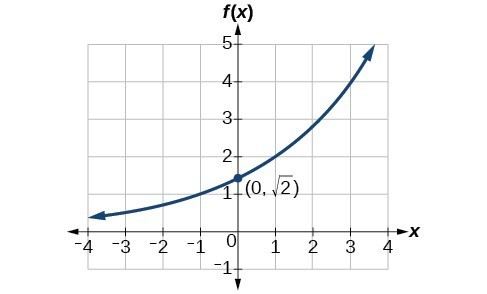Figure 6

Solution

How To: Given two points on the curve of an exponential function, use a graphing calculator to find the equation.

1. Press [STAT].
2. Clear any existing entries in columns L1 or L2.
3. In L1, enter the x-coordinates given.
4. In L2, enter the corresponding y-coordinates.
5. Press [STAT] again. Cursor right to CALC, scroll down to ExpReg (Exponential Regression), and press [ENTER].
6. The screen displays the values of a and b in the exponential equation $y=a\cdot {b}^{x}$.

Example 6: Using a Graphing Calculator to Find an Exponential Function

Use a graphing calculator to find the exponential equation that includes the points $\left(2,24.8\right)$ and $\left(5,198.4\right)$.

Solution

Follow the guidelines above. First press [STAT], [EDIT], [1: Edit…], and clear the lists L1 and L2. Next, in the L1 column, enter the x-coordinates, 2 and 5. Do the same in the L2 column for the y-coordinates, 24.8 and 198.4.

Now press [STAT], [CALC], [0: ExpReg] and press [ENTER]. The values = 6.2 and = 2 will be displayed. The exponential equation is $y=6.2\cdot {2}^{x}$.

Try It 6

Use a graphing calculator to find the exponential equation that includes the points (3, 75.98) and (6, 481.07).

Solution

Use Compound Interest Formulas

Savings instruments in which earnings are continually reinvested, such as mutual funds and retirement accounts, use compound interest. The term compounding refers to interest earned not only on the original value, but on the accumulated value of the account.

The annual percentage rate (APR) of an account, also called the nominal rate, is the yearly interest rate earned by an investment account. The term nominal is used when the compounding occurs a number of times other than once per year. In fact, when interest is compounded more than once a year, the effective interest rate ends up being greater than the nominal rate! This is a powerful tool for investing.

We can calculate the compound interest using the compound interest formula, which is an exponential function of the variables time t, principal P, APR r, and number of compounding periods in a year n:

$A\left(t\right)=P{\left(1+\frac{r}{n}\right)}^{nt}$

For example, observe the table below, which shows the result of investing $1,000 at 10% for one year. Notice how the value of the account increases as the compounding frequency increases. Frequency Value after 1 year Annually$1100
Semiannually $1102.50 Quarterly$1103.81
Monthly $1104.71 Daily$1105.16

A General Note: The Compound Interest Formula

Compound interest can be calculated using the formula

$A\left(t\right)=P{\left(1+\frac{r}{n}\right)}^{nt}$

where

• A(t) is the account value,
• t is measured in years,
• P is the starting amount of the account, often called the principal, or more generally present value,
• r is the annual percentage rate (APR) expressed as a decimal, and
• n is the number of compounding periods in one year.

If we invest $3,000 in an investment account paying 3% interest compounded quarterly, how much will the account be worth in 10 years? Solution Because we are starting with$3,000, = 3000. Our interest rate is 3%, so r = 0.03. Because we are compounding quarterly, we are compounding 4 times per year, so = 4. We want to know the value of the account in 10 years, so we are looking for A(10), the value when t = 10.

$\begin{cases}A\left(t\right)\hfill & =P\left(1+\frac{r}{n}\right)^{nt}\hfill & \text{Use the compound interest formula}. \\ A\left(10\right)\hfill & =3000\left(1+\frac{0.03}{4}\right)^{4\cdot 10}\hfill & \text{Substitute using given values}. \\ \text{ }\hfill & \approx 4045.05\hfill & \text{Round to two decimal places}.\end{cases}$

Solution

A 529 Plan is a college-savings plan that allows relatives to invest money to pay for a child’s future college tuition; the account grows tax-free. Lily wants to set up a 529 account for her new granddaughter and wants the account to grow to $40,000 over 18 years. She believes the account will earn 6% compounded semi-annually (twice a year). To the nearest dollar, how much will Lily need to invest in the account now? Solution The nominal interest rate is 6%, so = 0.06. Interest is compounded twice a year, so = 2. We want to find the initial investment, P, needed so that the value of the account will be worth$40,000 in 18 years. Substitute the given values into the compound interest formula, and solve for P.

$\begin{cases}A\left(t\right)\hfill & =P{\left(1+\frac{r}{n}\right)}^{nt}\hfill & \text{Use the compound interest formula}.\hfill \\ 40,000\hfill & =P{\left(1+\frac{0.06}{2}\right)}^{2\left(18\right)}\hfill & \text{Substitute using given values }A\text{, }r, n\text{, and }t.\hfill \\ 40,000\hfill & =P{\left(1.03\right)}^{36}\hfill & \text{Simplify}.\hfill \\ \frac{40,000}{{\left(1.03\right)}^{36}}\hfill & =P\hfill & \text{Isolate }P.\hfill \\ P\hfill & \approx 13,801\hfill & \text{Divide and round to the nearest dollar}.\hfill \end{cases}$

Lily will need to invest $13,801 to have$40,000 in 18 years.

Try It 8

Refer to Example 8. To the nearest dollar, how much would Lily need to invest if the account is compounded quarterly?

Solution

Evaluate exponential functions with base e

As we saw earlier, the amount earned on an account increases as the compounding frequency increases. The table below shows that the increase from annual to semi-annual compounding is larger than the increase from monthly to daily compounding. This might lead us to ask whether this pattern will continue.

Examine the value of $1 invested at 100% interest for 1 year, compounded at various frequencies. Frequency $A\left(t\right)={\left(1+\frac{1}{n}\right)}^{n}$ Value Annually ${\left(1+\frac{1}{1}\right)}^{1}$$2
Semiannually ${\left(1+\frac{1}{2}\right)}^{2}$ $2.25 Quarterly ${\left(1+\frac{1}{4}\right)}^{4}$$2.441406
Monthly ${\left(1+\frac{1}{12}\right)}^{12}$ $2.613035 Daily ${\left(1+\frac{1}{365}\right)}^{365}$$2.714567
Hourly ${\left(1+\frac{1}{\text{8766}}\right)}^{\text{8766}}$ $2.718127 Once per minute ${\left(1+\frac{1}{\text{525960}}\right)}^{\text{525960}}$$2.718279

Solution

Since the account is growing in value, this is a continuous compounding problem with growth rate = 0.10. The initial investment was $1,000, so = 1000. We use the continuous compounding formula to find the value after = 1 year: $\begin{cases}A\left(t\right)\hfill & =P{e}^{rt}\hfill & \text{Use the continuous compounding formula}.\hfill \\ \hfill & =1000{\left(e\right)}^{0.1} & \text{Substitute known values for }P, r,\text{ and }t.\hfill \\ \hfill & \approx 1105.17\hfill & \text{Use a calculator to approximate}.\hfill \end{cases}$ The account is worth$1,105.17 after one year.

A person invests $100,000 at a nominal 12% interest per year compounded continuously. What will be the value of the investment in 30 years? Solution Example 9: Calculating Continuous Decay Radon-222 decays at a continuous rate of 17.3% per day. How much will 100 mg of Radon-222 decay to in 3 days? Solution Since the substance is decaying, the rate, 17.3%, is negative. So, = –0.173. The initial amount of radon-222 was 100 mg, so = 100. We use the continuous decay formula to find the value after = 3 days: $\begin{cases}A\left(t\right)\hfill & =a{e}^{rt}\hfill & \text{Use the continuous growth formula}.\hfill \\ \hfill & =100{e}^{-0.173\left(3\right)} & \text{Substitute known values for }a, r,\text{ and }t.\hfill \\ \hfill & \approx 59.5115\hfill & \text{Use a calculator to approximate}.\hfill \end{cases}$ So 59.5115 mg of radon-222 will remain. Try It 11 Using the data in Example 9, how much radon-222 will remain after one year? Solution Key Equations  definition of the exponential function $f\left(x\right)={b}^{x}\text{, where }b>0, b\ne 1$ definition of exponential growth $f\left(x\right)=a{b}^{x},\text{ where }a>0,b>0,b\ne 1$ compound interest formula $\begin{cases}A\left(t\right)=P{\left(1+\frac{r}{n}\right)}^{nt} ,\text{ where}\hfill \\ A\left(t\right)\text{ is the account value at time }t\hfill \\ t\text{ is the number of years}\hfill \\ P\text{ is the initial investment, often called the principal}\hfill \\ r\text{ is the annual percentage rate (APR), or nominal rate}\hfill \\ n\text{ is the number of compounding periods in one year}\hfill \end{cases}$ continuous growth formula $A\left(t\right)=a{e}^{rt},\text{ where}$t is the number of unit time periods of growth a is the starting amount (in the continuous compounding formula a is replaced with P, the principal) e is the mathematical constant, $e\approx 2.718282$ Key Concepts • An exponential function is defined as a function with a positive constant other than 1 raised to a variable exponent. • A function is evaluated by solving at a specific value. • An exponential model can be found when the growth rate and initial value are known. • An exponential model can be found when the two data points from the model are known. • An exponential model can be found using two data points from the graph of the model. • An exponential model can be found using two data points from the graph and a calculator. • The value of an account at any time t can be calculated using the compound interest formula when the principal, annual interest rate, and compounding periods are known. • The initial investment of an account can be found using the compound interest formula when the value of the account, annual interest rate, compounding periods, and life span of the account are known. • The number e is a mathematical constant often used as the base of real world exponential growth and decay models. Its decimal approximation is $e\approx 2.718282$. • Scientific and graphing calculators have the key $\left[{e}^{x}\right]$ or $\left[\mathrm{exp}\left(x\right)\right]$ for calculating powers of e. • Continuous growth or decay models are exponential models that use e as the base. Continuous growth and decay models can be found when the initial value and growth or decay rate are known. Glossary annual percentage rate (APR) the yearly interest rate earned by an investment account, also called nominal rate compound interest interest earned on the total balance, not just the principal exponential growth a model that grows by a rate proportional to the amount present nominal rate the yearly interest rate earned by an investment account, also called annual percentage rate Section Exercises 1. Explain why the values of an increasing exponential function will eventually overtake the values of an increasing linear function. 2. Given a formula for an exponential function, is it possible to determine whether the function grows or decays exponentially just by looking at the formula? Explain. 3. The Oxford Dictionary defines the word nominal as a value that is “stated or expressed but not necessarily corresponding exactly to the real value.” Develop a reasonable argument for why the term nominal rate is used to describe the annual percentage rate of an investment account that compounds interest. For the following exercises, identify whether the statement represents an exponential function. Explain. 4. The average annual population increase of a pack of wolves is 25. 5. A population of bacteria decreases by a factor of $\frac{1}{8}$ every 24 hours. 6. The value of a coin collection has increased by 3.25% annually over the last 20 years. 7. For each training session, a personal trainer charges his clients$5 less than the previous training session.

8. The height of a projectile at time t is represented by the function $h\left(t\right)=-4.9{t}^{2}+18t+40$.

For the following exercises, consider this scenario: For each year t, the population of a forest of trees is represented by the function $A\left(t\right)=115{\left(1.025\right)}^{t}$. In a neighboring forest, the population of the same type of tree is represented by the function $B\left(t\right)=82{\left(1.029\right)}^{t}$. (Round answers to the nearest whole number.)

9. Which forest’s population is growing at a faster rate?

10. Which forest had a greater number of trees initially? By how many?

11. Assuming the population growth models continue to represent the growth of the forests, which forest will have a greater number of trees after 20 years? By how many?

12. Assuming the population growth models continue to represent the growth of the forests, which forest will have a greater number of trees after 100 years? By how many?

13. Discuss the above results from the previous four exercises. Assuming the population growth models continue to represent the growth of the forests, which forest will have the greater number of trees in the long run? Why? What are some factors that might influence the long-term validity of the exponential growth model?

For the following exercises, determine whether the equation represents exponential growth, exponential decay, or neither. Explain.

14. $y=300{\left(1-t\right)}^{5}$

15. $y=220{\left(1.06\right)}^{x}$

16. $y=16.5{\left(1.025\right)}^{\frac{1}{x}}$

17. $y=11,701{\left(0.97\right)}^{t}$

For the following exercises, find the formula for an exponential function that passes through the two points given.

18. $\left(0,6\right)$ and $\left(3,750\right)$

19. $\left(0,2000\right)$ and $\left(2,20\right)$

20. $\left(-1,\frac{3}{2}\right)$ and $\left(3,24\right)$

21. $\left(-2,6\right)$ and $\left(3,1\right)$

22. $\left(3,1\right)$ and $\left(5,4\right)$

For the following exercises, determine whether the table could represent a function that is linear, exponential, or neither. If it appears to be exponential, find a function that passes through the points.

23.

 x 1 2 3 4 f(x) 70 40 10 -20

24.

 x 1 2 3 4 h(x) 70 49 34.3 24.01

25.

 x 1 2 3 4 m(x) 80 61 42.9 25.61

26.

 x 1 2 3 4 f(x) 10 20 40 80

27.

 x 1 2 3 4 g(x) -3.25 2 7.25 12.5

For the following exercises, use the compound interest formula, $A\left(t\right)=P{\left(1+\frac{r}{n}\right)}^{nt}$.

28. After a certain number of years, the value of an investment account is represented by the equation $10,250{\left(1+\frac{0.04}{12}\right)}^{120}$. What is the value of the account?

29. What was the initial deposit made to the account in the previous exercise?

30. How many years had the account from the previous exercise been accumulating interest?

31. An account is opened with an initial deposit of $6,500 and earns 3.6% interest compounded semi-annually. What will the account be worth in 20 years? 32. How much more would the account in the previous exercise have been worth if the interest were compounding weekly? 33. Solve the compound interest formula for the principal, P. 34. Use the formula found in the previous exercise to calculate the initial deposit of an account that is worth$14,472.74 after earning 5.5% interest compounded monthly for 5 years. (Round to the nearest dollar.)

35. How much more would the account in the previous two exercises be worth if it were earning interest for 5 more years?

36. Use properties of rational exponents to solve the compound interest formula for the interest rate, r.

37. Use the formula found in the previous exercise to calculate the interest rate for an account that was compounded semi-annually, had an initial deposit of $9,000 and was worth$13,373.53 after 10 years.

38. Use the formula found in the previous exercise to calculate the interest rate for an account that was compounded monthly, had an initial deposit of $5,500, and was worth$38,455 after 30 years.

For the following exercises, determine whether the equation represents continuous growth, continuous decay, or neither. Explain.

39. $y=3742{\left(e\right)}^{0.75t}$

40. $y=150{\left(e\right)}^{\frac{3.25}{t}}$

41. $y=2.25{\left(e\right)}^{-2t}$

42. Suppose an investment account is opened with an initial deposit of $12,000 earning 7.2% interest compounded continuously. How much will the account be worth after 30 years? 43. How much less would the account from Exercise 42 be worth after 30 years if it were compounded monthly instead? For the following exercises, evaluate each function. Round answers to four decimal places, if necessary. 44. $f\left(x\right)=2{\left(5\right)}^{x}$, for $f\left(-3\right)$ 45. $f\left(x\right)=-{4}^{2x+3}$, for $f\left(-1\right)$ 46. $f\left(x\right)={e}^{x}$, for $f\left(3\right)$ 47. $f\left(x\right)=-2{e}^{x - 1}$, for $f\left(-1\right)$ 48. $f\left(x\right)=2.7{\left(4\right)}^{-x+1}+1.5$, for $f\left(-2\right)$ 49. $f\left(x\right)=1.2{e}^{2x}-0.3$, for $f\left(3\right)$ 50. $f\left(x\right)=-\frac{3}{2}{\left(3\right)}^{-x}+\frac{3}{2}$, for $f\left(2\right)$ For the following exercises, use a graphing calculator to find the equation of an exponential function given the points on the curve. 51. $\left(0,3\right)$ and $\left(3,375\right)$ 52. $\left(3,222.62\right)$ and $\left(10,77.456\right)$ 53. $\left(20,29.495\right)$ and $\left(150,730.89\right)$ 54. $\left(5,2.909\right)$ and $\left(13,0.005\right)$ 55. $\left(11,310.035\right)$ and $\left(25,356.3652\right)$ 56. The annual percentage yield (APY) of an investment account is a representation of the actual interest rate earned on a compounding account. It is based on a compounding period of one year. Show that the APY of an account that compounds monthly can be found with the formula $\text{APY}={\left(1+\frac{r}{12}\right)}^{12}-1$. 57. Repeat the previous exercise to find the formula for the APY of an account that compounds daily. Use the results from this and the previous exercise to develop a function $I\left(n\right)$ for the APY of any account that compounds n times per year. 58. Recall that an exponential function is any equation written in the form $f\left(x\right)=a\cdot {b}^{x}$ such that a and b are positive numbers and $b\ne 1$. Any positive number b can be written as $b={e}^{n}$ for some value of n. Use this fact to rewrite the formula for an exponential function that uses the number as a base. 59. In an exponential decay function, the base of the exponent is a value between 0 and 1. Thus, for some number $b>1$, the exponential decay function can be written as $f\left(x\right)=a\cdot {\left(\frac{1}{b}\right)}^{x}$. Use this formula, along with the fact that $b={e}^{n}$, to show that an exponential decay function takes the form $f\left(x\right)=a{\left(e\right)}^{-nx}$ for some positive number n. 60. The formula for the amount A in an investment account with a nominal interest rate r at any time t is given by $A\left(t\right)=a{\left(e\right)}^{rt}$, where a is the amount of principal initially deposited into an account that compounds continuously. Prove that the percentage of interest earned to principal at any time t can be calculated with the formula $I\left(t\right)={e}^{rt}-1$. 61. The fox population in a certain region has an annual growth rate of 9% per year. In the year 2012, there were 23,900 fox counted in the area. What is the fox population predicted to be in the year 2020? 62. A scientist begins with 100 milligrams of a radioactive substance that decays exponentially. After 35 hours, 50mg of the substance remains. How many milligrams will remain after 54 hours? 63. In the year 1985, a house was valued at$110,000. By the year 2005, the value had appreciated to $145,000. What was the annual growth rate between 1985 and 2005? Assume that the value continued to grow by the same percentage. What was the value of the house in the year 2010? 64. A car was valued at$38,000 in the year 2007. By 2013, the value had depreciated to $11,000 If the car’s value continues to drop by the same percentage, what will it be worth by 2017? 65. Jamal wants to save$54,000 for a down payment on a home. How much will he need to invest in an account with 8.2% APR, compounding daily, in order to reach his goal in 5 years?

66. Kyoko has $10,000 that she wants to invest. Her bank has several investment accounts to choose from, all compounding daily. Her goal is to have$15,000 by the time she finishes graduate school in 6 years. To the nearest hundredth of a percent, what should her minimum annual interest rate be in order to reach her goal? (Hint: solve the compound interest formula for the interest rate.)

67. Alyssa opened a retirement account with 7.25% APR in the year 2000. Her initial deposit was $13,500. How much will the account be worth in 2025 if interest compounds monthly? How much more would she make if interest compounded continuously? 68. An investment account with an annual interest rate of 7% was opened with an initial deposit of$4,000 Compare the values of the account after 9 years when the interest is compounded annually, quarterly, monthly, and continuously.

1. Todar, PhD, Kenneth. Todar's Online Textbook of Bacteriology. http://textbookofbacteriology.net/growth_3.html.
2. http://www.worldometers.info/world-population/. Accessed February 24, 2014.
3. Oxford Dictionary. http://oxforddictionaries.com/us/definition/american_english/nomina.